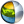Cg 3.1 Toolkit Documentation Cg / Standard Library / round

## Name

round - returns the rounded value of scalars or vectors

## Synopsis

```float  round(float  a);
float1 round(float1 a);
float2 round(float2 a);
float3 round(float3 a);
float4 round(float4 a);

half   round(half  a);
half1  round(half1 a);
half2  round(half2 a);
half3  round(half3 a);
half4  round(half4 a);

fixed  round(fixed  a);
fixed1 round(fixed1 a);
fixed2 round(fixed2 a);
fixed3 round(fixed3 a);
fixed4 round(fixed4 a);
```

## Parameters

a
Scalar or vector.

## Description

Returns the rounded value of a scalar or vector.

For vectors, the returned vector contains the rounded value of each element of the input vector.

The round operation returns the nearest integer to the operand. The value returned by round() if the fractional portion of the operand is 0.5 is profile dependent. On older profiles without built-in round() support, round-to-nearest up rounding is used. On profiles newer than fp40/vp40, round-to-nearest even is used.

## Reference Implementation

round for float could be implemented this way:

```// round-to-nearest even profiles
float round(float a)
{
float x = a + 0.5;
float f = floor(x);
float r;
if (x == f) {
if (a > 0)
r = f - fmod(f, 2);
else
r = f + fmod(f, 2);
} else
r = f;
return r;
}

// round-to-nearest up profiles
float round(float a)
{
return floor(x + 0.5);
}
```

## Profile Support

round is supported in all profiles except fp20.| Cg Toolkit | Download | Release Archive | Profiles | Reference | Books | Discussions |